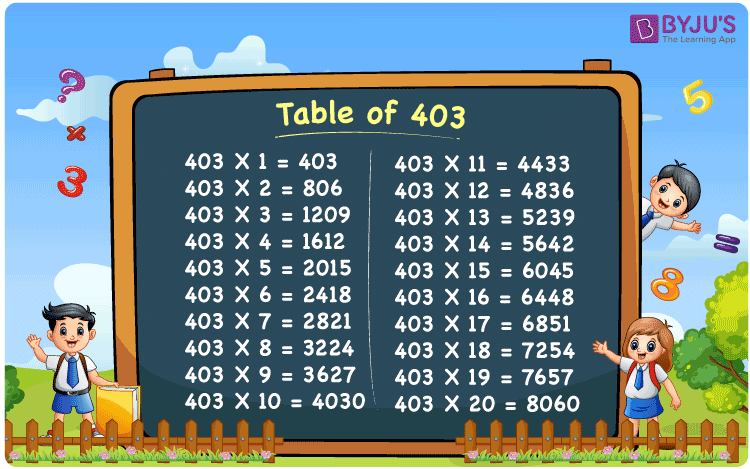Checkout JEE MAINS 2022 Question Paper Analysis : Checkout JEE MAINS 2022 Question Paper Analysis :

# Table of 403

The table of 403 is the multiplication of 403 by natural numbers, i.e. 403 × 1 = 403, 403 × 2 = 806, 403 × 3 = 1209, 403 × 4 = 1612, etc. As we know, learning the multiplication tables helps to solve multiplication-related problems quickly and accurately, which will help you save time in calculations. Here, you will learn one of the maths tables called the 403 times table. Students are advised to download the PDF of the table of 403 to learn both online and offline.

## Table of 403 Chart## What is the 403 Times Table?

In maths, the 403 times table can be created using two simple arithmetic operations, addition and multiplication. The below table shows the table of 403 up to 10 results that are generated from the multiplication of 403 by numbers from 1 to 10, and using repeated addition.

 Using multiplication Using repeated addition 403 × 1 = 403 403 403 × 2 = 806 403 + 403 = 806 403 × 3 = 1209 403 + 403 + 403 = 1209 403 × 4 = 1612 403 + 403 + 403 + 403 = 1612 403 × 5 = 2015 403 + 403 + 403 + 403 + 403 = 2015 403 × 6 = 2418 403 + 403 + 403 + 403 + 403 + 403 = 2418 403 × 7 = 2821 403 + 403 + 403 + 403 + 403 + 403 + 403 = 2821 403 × 8 = 3224 403 + 403 + 403 + 403 + 403 + 403 + 403 + 403 = 3224 403 × 9 = 3627 403 + 403 + 403 + 403 + 403 + 403 + 403 + 403 + 403 = 3627 403 × 10 = 4030 403 + 403 + 403 + 403 + 403 + 403 + 403 + 403 + 403 + 403 = 4030

## Multiplication Table of 403

Go through the multiplication table of 403 given below, which shows the multiplication of 403 up to 20 times.

 403 × 1 = 403 403 × 2 = 806 403 × 3 = 1209 403 × 4 = 1612 403 × 5 = 2015 403 × 6 = 2418 403 × 7 = 2821 403 × 8 = 3224 403 × 9 = 3627 403 × 10 = 4030 403 × 11 = 4433 403 × 12 = 4836 403 × 13 = 5239 403 × 14 = 5642 403 × 15 = 6045 403 × 16 = 6448 403 × 17 = 6851 403 × 18 = 7254 403 × 19 = 7657 403 × 20 = 8060

## Example on the Table of 403

Question:

Riya sleeps 8 hours every day. How much time did she spend on sleep in 403 days?

Solution:

Number of hours slept by Riya in a day = 8

Number of days = 403

Total time spent on sleeping = 403 × 8 = 3224 hours.

## Frequently Asked Questions on Table of 403

### What is the table of 403?

The table of 403 is also called the multiplication table of 403 or the 403 times table. We can write the table of 403 as 403 × 1 = 403, 403 × 2 = 806, 403 × 3 = 1209, 403 × 4 = 1612, 403 × 5 = 2015, and so on.

### Does 403 come in any table?

Yes, 403 comes in the 13 times table. When 13 is multiplied by 31, we get 403, i.e. 13 times 31 = 13 × 31 = 403.

### How many times of 403 is 6448?

Sixteen times of 403 or 403 times 16 is 6448, i.e. 403 × 16 = 6448.